# Discriminative Embeddings of Latent Variable Models for Structured Data(structure2vec)阅读笔记

## 网络嵌入

Posted by Yunlongs on March 21, 2020

## Discriminative Embeddings of Latent Variable Models for Structured Data(structure2vec)

### 前言

structure2vec算法和其他kernel 消息传递的算法主要不同点在于：

1. 可以学习结构化数据的相似性度量。
2. 使用判别信息来学习非线性映射
3. can run in a mean field update fashion（没太懂）

### 背景

kernel 方法： 假设结构化数据被表示为$\chi \in \mathcal{G}$，kernel函数为$k\left(\chi, \chi^{\prime}\right): \mathcal{G} \times \mathcal{G} \mapsto \mathbb{R}$，并且kernel矩阵需要为半正定矩阵。kernel方法的一个特点是，可以在不需要访问原数据点来学习映射的情况下，直接使用其kenel值在高维空间中工作。

kernel for structured data: 每个kernel函数都等同于一些特征映射$\phi(\chi)$，其中kernel函数可以被表达成特征映射间内积的形式，比如$k\left(\chi, \chi^{\prime}\right)=\left\langle\phi(\chi), \phi\left(\chi^{\prime}\right)\right\rangle$。

$k\left(\chi, \chi^{\prime}\right)=\sum_{s \in \mathcal{S}} \#(s \in \chi) \#\left(s \in \chi^{\prime}\right)$

Hilbert Space Embedding of Distributions: Hilbert空间嵌入是将分布映射到潜在的无限维特征空间中：

$\mu_{X}:=\mathbb{E}_ {X}[\phi(X)]=\int_{\mathcal{X}} \phi(x) p(x) d x: \mathcal{P} \mapsto \mathcal{F}$

$f(p(x))=\tilde{f}\left(\mu_{X}\right)$

### 3. Model for a Structured Data Point

$p\left(\left\lbrace H_{i}\right\rbrace ,\left\lbrace X_{i}\right\rbrace \right) \propto \prod_{i \in \mathcal{V}} \Phi\left(H_{i}, X_{i}\right) \prod_{(i, j) \in \mathcal{E}} \Psi\left(H_{i}, H_{j}\right)$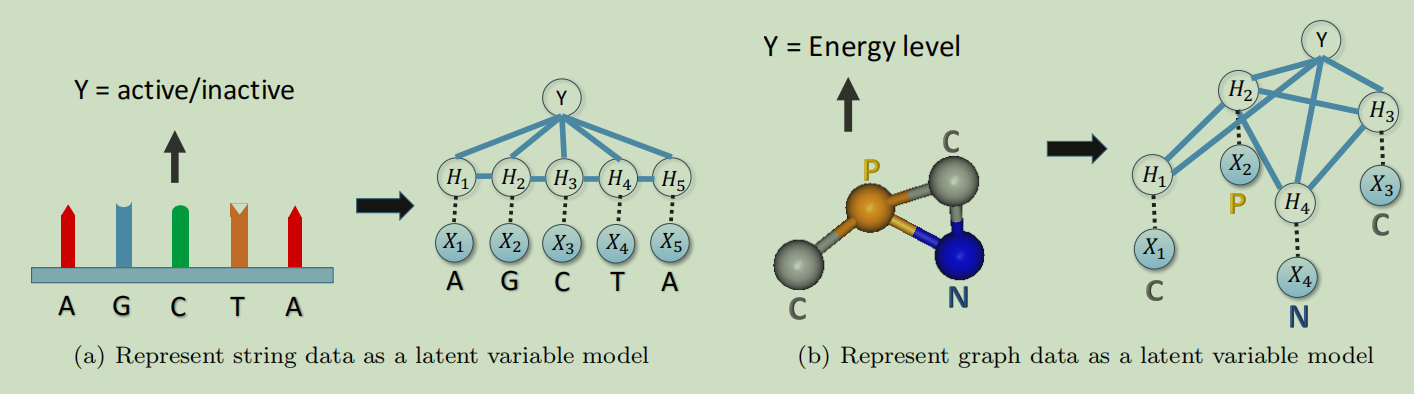### 4.Embedding Latent Variable Models

$\mu_{i}=\int_{\mathcal{H}} \phi\left(h_{i}\right) p\left(h_{i} \|\left\lbrace x_{i}\right\rbrace \right) d h_{i}$

$\phi\left(H_{i}\right)$和$\operatorname{MRF} p\left(H_{i} |\left\lbrace x_{i}\right\rbrace \right)$中参数的精确形式在当前是不固定的， 我们将在稍后使用监督信号来学习它们作为最终判别目标。现在我们假设$\phi\left(H_{i}\right) \in \mathbb{R}^{d}$在一个有限维的特征空间中，并且精确的$d$值将会在之后的实验中通过交叉验证来确定。

$p\left(H_{i} |\left\lbrace x_{i}\right\rbrace \right)=\int_{\mathcal{H}^{V-1}} p\left(H_{i},\left\lbrace h_{j}\right\rbrace |\left\lbrace x_{j}\right\rbrace \right) \prod_{j \in \mathcal{V} \backslash i} d h_{j}$

### 4.1 Embedding Mean-Field Inference

vanilla mean-field inference试图去使用独立密度成分的乘积来去近似$p\left(\left\lbrace H_{i}\right\rbrace |\left\lbrace x_{i}\right\rbrace \right)$，其中$q_{i}\left(h_{i}\right) \geqslant 0$是有效密度函数，并且$\int_{\mathcal{H}} q_{i}\left(h_{i}\right) d h_{i}=1$。

$\min _{q_{1}, \ldots, q_{d}} \int_{\mathcal{H}^{d}} \prod_{i \in \mathcal{V}} q_{i}\left(h_{i}\right) \log \frac{\prod_{i \in \mathcal{V}} q_{i}\left(h_{i}\right)}{p\left(\left\lbrace h_{i}\right\rbrace |\left\lbrace x_{i}\right\rbrace \right)} \prod_{i \in \mathcal{V}} d h_{i}$

\begin{aligned} \log q_{i}\left(h_{i}\right) &=c_{i}+\log \left(\Phi\left(h_{i}, x_{i}\right)\right)+\sum_{j \in \mathcal{N}(i)} \int_{\mathcal{H}} q_{j}\left(h_{j}\right) \log \left(\Psi\left(h_{i}, h_{j}\right) \Phi\left(h_{j}, x_{j}\right)\right) d h_{j} \\ &=c_{i}^{\prime}+\log \Phi\left(h_{i}, x_{i}\right)+\sum_{j \in \mathcal{N}(i)} \int_{\mathcal{H}} q_{j}\left(h_{j}\right) \log \Psi\left(h_{i}, h_{j}\right) d h_{j} \end{aligned}

$q_{i}\left(h_{i}\right)=f\left(h_{i}, x_{i},\left\lbrace q_{j}\right\rbrace _{j \in \mathcal{N}(i)}\right)$

$\widetilde{\mu}_{i}=\int_{\mathcal{H}} \phi\left(h_{i}\right) q_{i}\left(h_{i}\right) d h_{i}$

$\tilde{\mu}_ {i}=\tilde{\mathcal{T}} \circ\left(x_{i},\left\lbrace \tilde{\mu}_ {j}\right\rbrace _{j \in \mathcal{N}(i)}\right)$

$\tilde{\mu}_ {i}=\sigma\left(W_{1} x_{i}+W_{2} \sum_{j \in \mathcal{N}(i)} \tilde{\mu}_{j}\right)$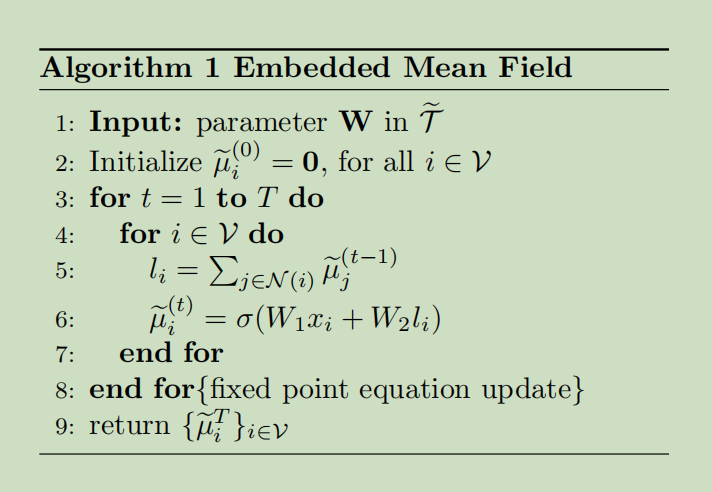### 4.2 Embedding Loopy Belief Propagation

Loopy belief propagation是另一个变分推断方法，其本质上通过考虑成对的关系来优化Bethe free energy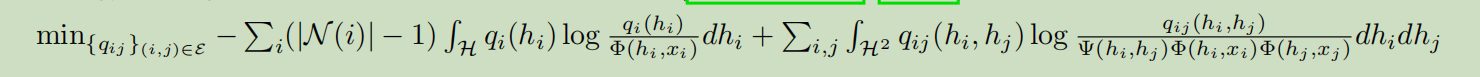其服从pairwise marginal一直约束：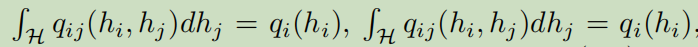并且$\int_{\mathcal{H}} q_{i}\left(h_{i}\right) d h_{i}=1$。对于所有的$(i,j)\in mathcal E$,我们可以得到上面优化问题的所有不动点条件：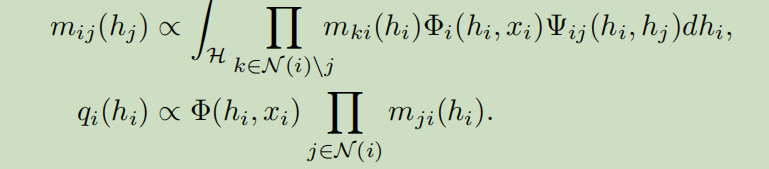其中$m_{ij}(h_j)$是从节点i到j的中间结果，另外，$m_{ij}(h_j)$是一个非负函数，所以其也可以被标准化为一个密度函数，因此也可以被embeded。

### 5. Discriminative Training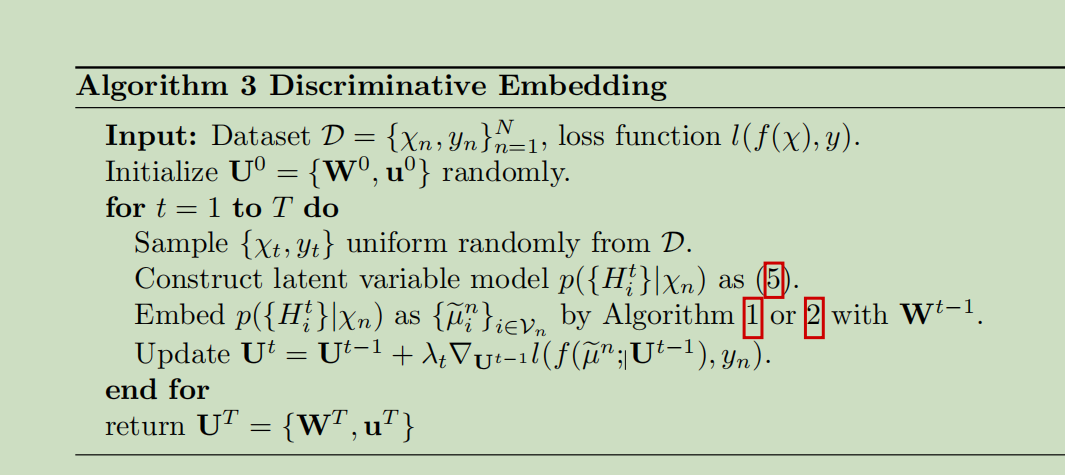### 6.实验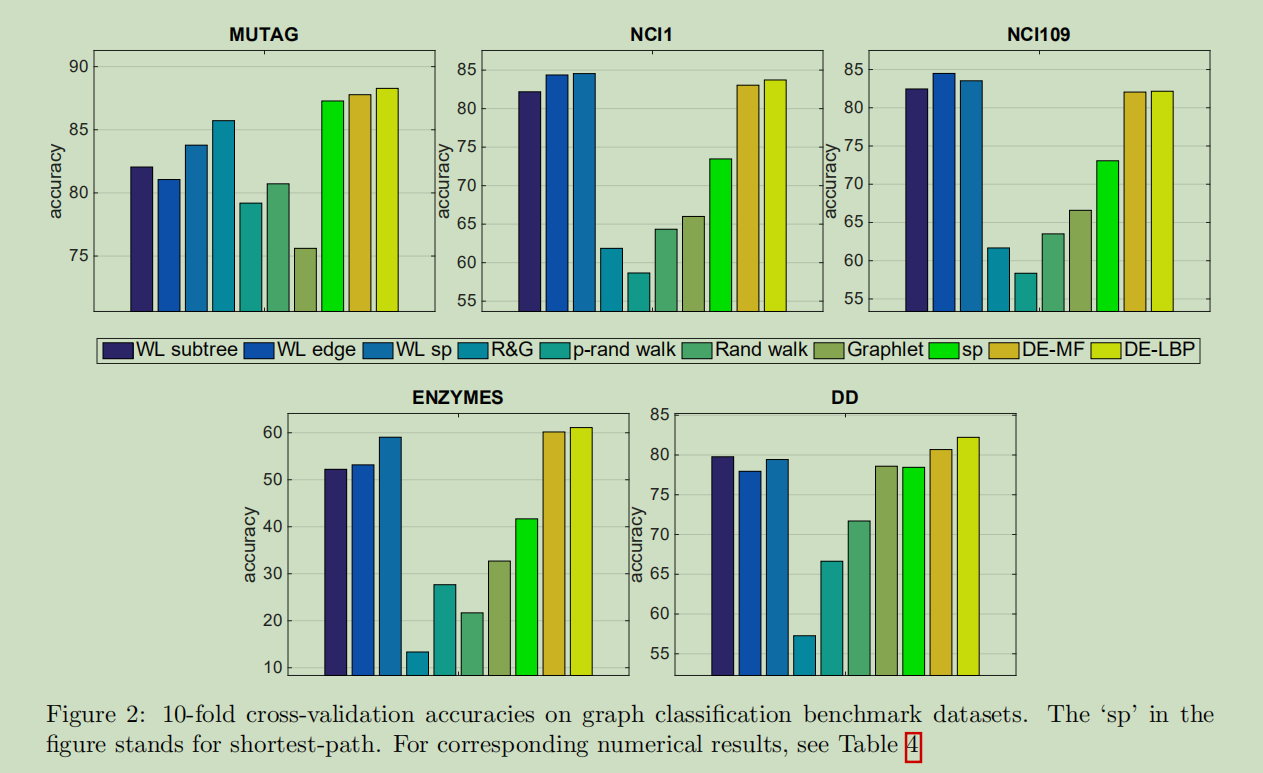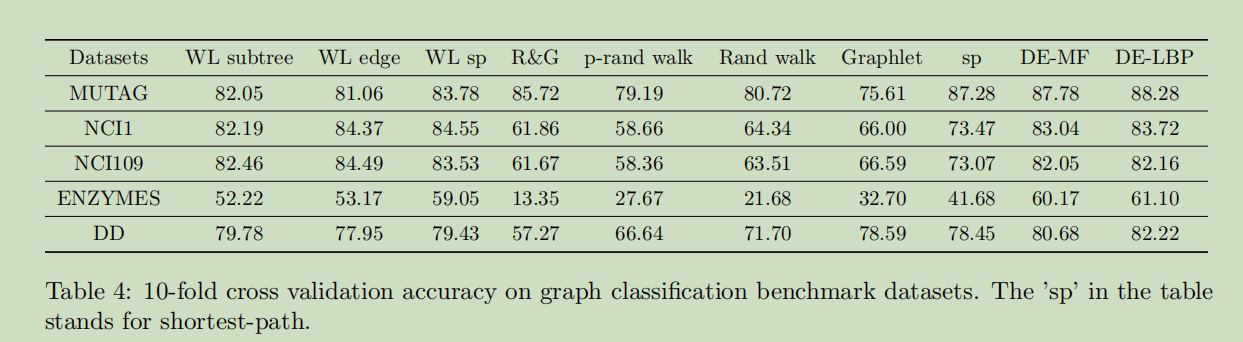可以看到其准确度相较于以前的方法还是有较大的提升的。